Support for General Recursion

In this lecture, we shall consider the support for the general case of recursion.

We shall also restrict the implementation somewhat in order to
illustrate the important points without “going overboard” on the complexities.

In FORTRAN terms, there are two classes of procedures.

Functions        Those procedures that return a value and, in the ideal case, have
no effect on the calling program other than passing that value.

Subroutines     Those procedures that perform general actions and optionally
can alter a number of variables in the calling program.

In this lecture, everything will be called a procedure.

The issues to be addressed in providing for recursion are as follows:

2.   Passing the arguments to the procedure.

3.   Handling variables declared locally in the procedure, whose scope does not extend
beyond that procedure; they cannot be used directly in the calling program.

Outline of the Lecture

We shall cover the topics in roughly the sequence below.

1.   The assumptions defining the context in which recursion is used.

2.   The idea of generalizing the stack to provide efficient support for recursion.

3.   Allocation of areas of the stack for arguments to the procedure and
variables local to the procedure.

4.   Declaration of static variables with scope local to the procedure.  In Java, these
may be called class variables.

5.   Call by value and call by reference.  Writing code to access each of these
as stored on the stack, and how to modify arguments passed by reference.

6.   A few modest proposals for increasing the security of the run–time system,
and consideration of the difficulties in their implementation.

Assumption 1: The Linkage Code is Consistent

The high–level description of the procedure linkage problem is as follows.

1.   The calling program invokes the procedure.

a)   It places the return address on the stack.

b)   It places the arguments on the stack.

2.   The called procedure receives control.

a)   It modifies the stack to create room for its variables with local scope.

b)   It accesses the arguments and uses them as specified.

Thus we must have two sections of code that must be consistent.  Design of the
calling code will force the design of the receiving code.

In particular, the handling of arguments passed by value must be different from
that for arguments passed by reference.

In this lecture, my assumption is that the assembly language I write for each section
will be emitted by a well–designed compiler, so that consistency is enforced.

Assumption 2: The Executable Code is Loaded Statically

The assumption here is that each block of code (main program, subroutine, function, etc.)
during the execution of the program.

Variables corresponding to data declarations within a procedure are static.

Consider the following code, which uses a number of externally declared labels.

It might be modified into a print routine that records the count of pages printed.

A10LOOP  MVC DATAPR,RECORDIN          MOVE INPUT RECORD

LH  R7,PAGENUM               LOAD THE OLD PAGE NUMBER

AH  R7,=H‘1’                 INCREMENT BY 1

STH R7,PAGENUM               SAVE THE NEW COUNT

PUT PRINTER,PRINT            PRINT THE RECORD

BR  R8                       R8 HOLDS RETURN ADDRESS

PAGENUM  DC  H‘0’                     THE PAGE COUNTER

The label PAGENUM, as used above, should be considered a local variable that is
declared statically.  There are two interesting features of such variables.

1.   It will retain its value across invocations.

2.   All instances of the procedure access the same location when accessing
the value denoted by
PAGENUM.

The Memory Map for Static Allocation

Here is a sample memory layout for a MAIN program and a procedure PROC1.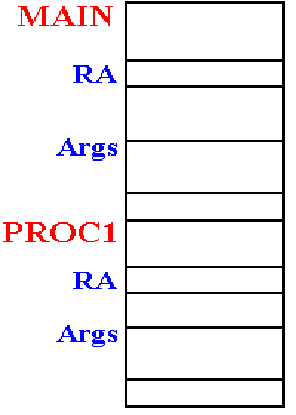This is to be considered a map of the computer
memory at the time this program is executing.

The data areas that are labeled as “ARGS” are
statically allocated.

Each reference to a label in one of these refers to
the same address in computer memory.

This arrangement will not work for recursion, even if
the return addresses are handled by a stack.

The Problem with Static Allocation

Consider the following code fragments related to the factorial program.

It is easier to see this problem when DOFACT is written in a higher level language.

Here is a plausible implementation.

Integer DoFact (Integer N) ;

var M : Integer ; // A local variable, declared static

Begin

If (N <= 1) Then DoFact := 1

Else

Begin

M = N;    // Save the current value

DoFact := M * DoFact(N – 1);

End

End

If the storage allocation for M is on the stack, this will work well.

If the storage allocation for M is static, this fails.

Static Allocation: An Execution Trace

Suppose that the label M is statically allocated.  Here is an execution trace.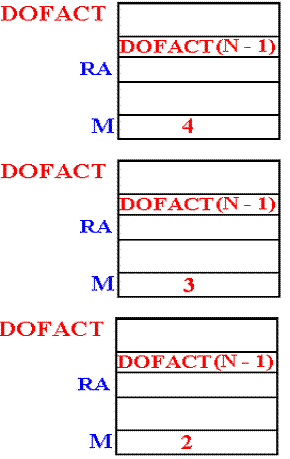DOFACT (4) is called and begins execution.

The value 4 is stored in the location associated with
label M.  DOFACT (3) is called

DOFACT (3) begins execution.

The value 3 is stored in the location associated with
label M, overwriting the previous value.

DOFACT (2) begins execution

The value 2 is stored in the location associated with
label M, overwriting the previous value.

Assume that DOFACT (1) does not store a value.

Our result is FACT(4) = 2·FACT(3) = 2·2·FACT(2) = 2·2·2·FACT(1) = 2·2·2 = 8.

Assumption 3: No Use of Global Variables

The problem of global variables is illustrated by the following code fragment,
which is written in a variant of PASCAL.

Program Main;

var X, Y : Real ;

Procedure Sub_1;

var Y, Z : Integer ;

Begin     // Body of Sub_1

End ;

Begin       // Body of main.

End ;

Within procedure MAIN, variables X and Y are defined.  Variable Z, being local
to procedure Sub_1, is not visible within MAIN.

Within procedure Sub_1, variables X, Y, and Z all have meaning, though the
variable Y denotes the local copy.

The problem is that of providing procedure Sub_1 with a reference to global variable X,
if it is not passed as an argument.  While this is easy, I choose to ignore this issue.

Stack Support for Generalized Recursion

In this lecture, I shall define one plausible stack organization to support recursive
procedures with arguments and local variables.

Again, I shall not consider the problem of access to global variables.

The structure of the stack is dictated by how it is used in the context of calling.

1.   A procedure will be executing.  It accesses all of its local variables via the stack.

At this point, this procedure calls another.

2.   Each of the arguments is pushed onto the stack.

3.   The return address is pushed onto the stack.

4.   The new procedure is invoked.

5.   The new procedure allocates space on the stack for its local variables.

This sequence dictates the structure of the stack.

That part of the stack directly used by a procedure is called an “activation record”.

Implied Structure of the Stack

For each procedure, here is the form of the activation record, as seen on the stack.

I should first note that there are many ways to structure the activation record, this is
just my way that I find easiest for presentation to this class.

Here is the form of the stack upon entry to a procedure.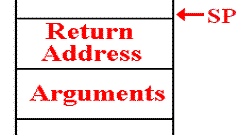Here is the form of the stack after the procedure has allocated space for local variables.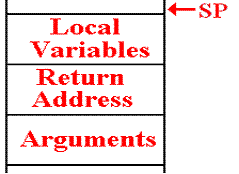A New Way to Access the Stack

At this point, I restate the design decision that only 32–bit fullwords are placed
onto the stack.  Effectively, this limit values to four types.

1.   The contents of a general purpose register.

2.   The contents of a 32–bit fullword.

3.   The contents of a 16–bit halfword, sign extended to a fullword.

4.   Any address, treated as a 32–bit fullword.

The traditional stack structure is accessed one item at a time using only PUSH and
POP operations.  For this use, it will be necessary to devise new stack operations.

Block allocation will be used to reserve a number of slots in the stack for use in
either passing arguments or in providing space for local variables.

Block deallocation will be used to move the stack pointer without necessarily
popping the values from the stack.

Block deallocation is used on return from a procedure.  In essence, we just
change the value of the stack pointer directly.

A Slight Redefinition of the Stack

In my earlier work on a stack, I had become interested in an Abstract Data Type definition, in which the value of the SP (Stack Pointer) is stored in the structure.

I now find it useful to allocate a register as the SP.

Here are two code fragments that show the new definition of the stack.

Here are some equates that define new uses for general purpose registers.

RVAL    EQU 3     // FUNCTION RETURN VALUE

SP      EQU 4     // STACK POINTER

FP      EQU 5     // ANOTHER USEFUL POINTER.

The stack itself is now just an allocation of memory.  Let’s make it bigger.

THESTACK DC 512F’0’  THE STACK NOW HOLDS 512 FULLWORDS

We still require some code to initialize the stack.  The key code might be

Sample Procedure

First, I want to postulate a recursive subroutine and describe its key features in
a pseudo–language.  Since I do not know what it does, it is called
DOWHAT.

Recall that I prefer UPPER CASE letters, as I find them easier to read.

Here is the essential declaration.

PROCEDURE DOWHAT ( INT X  ;   // PASSED BY REFERENCE

INT Y  ;   // PASSED BY VALUE

INT Z  ) ; // PASSED BY VALUE

// LOCAL VARIABLES

INT L1, L2, L3, L4

Recall the argument passing mechanisms.

It would make sense to have code such as X = Y + Z; the value of X changes.

One could write code such as Y = X + Z; this would have effect only in DOWHAT.

Conventions for Argument Handling

Recall that the goal of the compiler designer is to convert a high–level language statement into a sequence of assembly language statements having the same effect.

The requirement is to place the arguments onto the stack.

For this, it will be convenient to use a standard STKPUSH.

What is the sequence of pushing the arguments?  Is it X, Y, Z or Z, Y, X?

Are they pushed right to left or left to right.  All that matters is consistency.

Arbitrary choices:

1.   I shall push the arguments right to left.

2.   Because I find it interesting, I shall also push the argument count.

PUSH (Value of Z)     Called by value

PUSH (Value of Y)     Called by value

PUSH (Address of X)   Called by reference

PUSH (3)              Three arguments passed.

Calling DOWHAT

For now, we assume that locations X, Y, and Z have declarations of the type.

X        DC   F‘0’

Y        DC   F‘0’

Z        DC   F‘0’

Here is the code involved in an invocation of DOWHAT.

STKPUSH Z        Push value of Z

STKPUSH Y        Push value of Y

STKPUSH X,A      Push address of X

STKPUSH =F‘3’    Push the constant value 3

STKPUSH A1,A     Push the return address

B DOWHAT         Now call the procedure.

A1       Return Address: this code next.

My preference here is to use the explicit push operation, rather than a

The State of the Stack

We consider the state of the stack at this point.  It can be at one of two
equivalent points.

1.   Just before DOWHAT has been called.

2.   Just after DOWHAT has been called, but before any of its code has executed.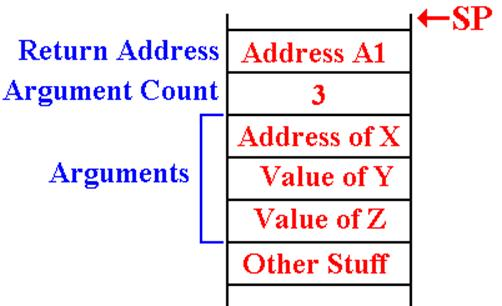DOWHAT Allocates Its Local Variables

At this point, I wish to introduce a FP (Frame Pointer) and warn that my use of
this is almost certainly to be non–standard and have flaws that are not apparent to me.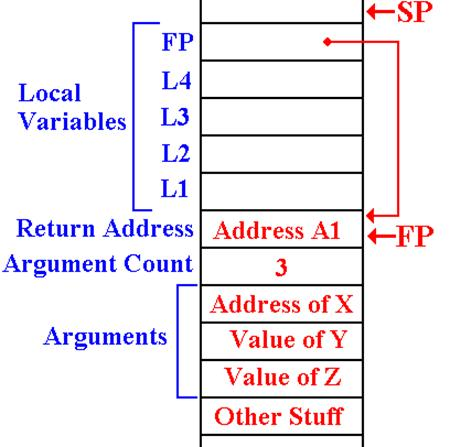The four local variables are allocated on the stack.

Here, I arbitrarily set the FP to indicate the location of the return address.

I also elect to store the current value of FP as a local variable.

This is the status of the stack during any execution of DOWHAT.

Entry Code for DOWHAT

The entry code for DOWHAT is the code to allocate the local variables, define
the value of the FP, and store a local copy for later use.

For four local variables we want to allocate five locations on the stack.
Here is the situation on entry.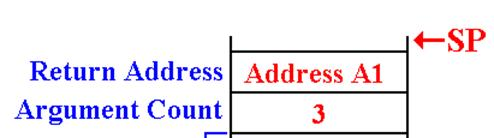Here is the code to allocate the local variables.

LR FP,SP        // SET UP THE FRAME POINTER

SH FP,=H‘4’     // IT NOW POINTS TO THE RETURN ADDR.

AH SP,=H‘20’    // MOVE THE STACK POINTER

ST FP,20(0,FP)  // SAVE THE LOCAL COPY

DOWHAT Accesses Its Arguments

Recall the status of the stack when DOWHAT is called.  Here, I ignore the local
variables and focus on the arguments.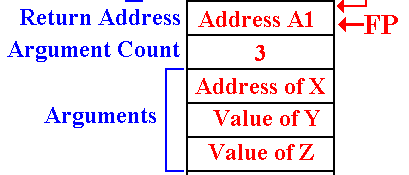The value of the first argument will be accessed somewhat as follows.

To get the value:     L  R8,–8(0,FP)  // Get the address

L  R9,0(0,R8)   // Get the value

To store a value:     L  R8,–8(0,FP)  // Get the address

ST R9,0(0,R8)   // Store the new value

Others are accessed by value, as in L  R9,–12(0,FP).

DOWHAT Returns

For the moment, I skip what the routine might do and indicate how it returns.

The first step in a return is to de–allocate the local variables.  This is easily
done by giving the stack pointer a new value.  Here is the code

LR SP,FP       // Change the value and thus remove

LR R9,-4(0,SP) // Get the argument count

AH R9,=H‘1’    // Add 1 to the value.

SLA R9,2       // Multiply by 4; get byte offset

SR  SP,R9      // Adjust stack pointer.  It now
// points to the first word allocated
// for this invocation of DOWHAT.

LR R9,0(0,FP)  // Get the return address

DOWHAT Calls Itself

Suppose that DOWHAT calls itself recursively with something like

DOWHAT (L1, L2, L3)

A2       Next instruction in DOWHAT

How do we handle the call and return?

First, we need to look at the stack at the time that DOWHAT calls itself.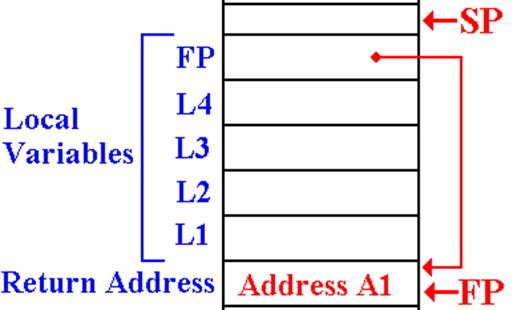Recall that L3 is pushed first.
The code for this might be .

STKPUSH 12,(0,FP)

STKPUSH 8,(0,FP)

STKPUSH 4,(0,FP),A

LH R9,=H‘3’

STKPUSH R9,R

STKPUSH A2,A

B DOWHAT

DOWHAT Processes a Return

Here we consider a return from another procedure, perhaps a recursive call.

Consider the code just above.

DOWHAT (L1, L2, L3)

A2       Next instruction in DOWHAT

What happens at address A2?  Recall the status of the “top part” of the stack.
All we do is to locate where the FP is stored and restore its value.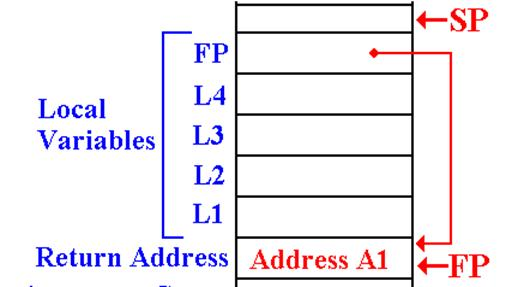L FP,-4(0,SP)

We have now returned to the
execution environment
appropriate for this
invocation of DOWHAT.